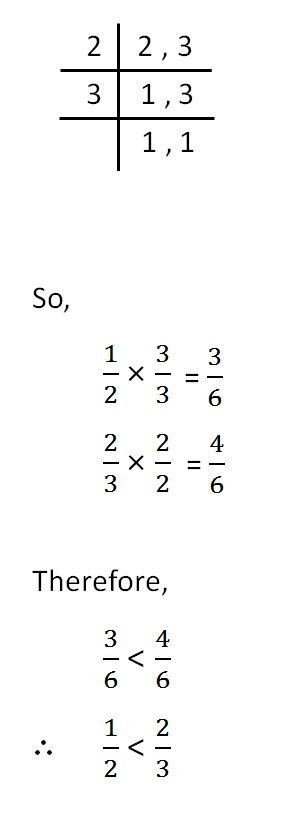Rational numbers greater or smaller

Chapter 1 Class 8 Rational Numbers
Concept wise

We can have 3 cases

1. Both numbers are positive
2. Both numbers are negative
3. One is positive, one is negative

Let’s look at each of them separately

## Both numbers are positive

2/3 & 4/3

Here, denominator is same.

So, we compare numerator.

2 < 4

∴ 2/3 < 4/3

1/2 & 2/3

First of all, we make denominator same

Common denominator = LCM of 2 & 3

= 2 × 3

= 6

## Both numbers are negative

When both numbers are negative,

we ignore the signs & compare

And then we reverse the order

(-2)/3 & (-4)/3

Ignoring signs

2/3 & 4/3

Here, denominator is same.

So, we compare numerator.

2 < 4

∴ 2/3 < 4/3

Multiplying −1 both sides

2/3 × −1 > 4/3 × −1

(-2)/3 > (-4)/3

(- 1 )/ 2 & (- 2 )/3

Ignoring signs

1/2 & 2/3

Making denominator same

Common denominator = LCM of 2 & 3

= 2 × 3

= 6Multiplying −1 both sides

1/2 × −1 > 2/3 × −1

(-1)/2 > (-2)/3

## One number is negative, one is positive

The negative number is always smaller

(-2)/3 & 4/3

(-2)/3 < 4/3

And,

1/2 & 2/(-3)

1/2 > 2/(-3)

Learn in your speed, with individual attention - Teachoo Maths 1-on-1 Class